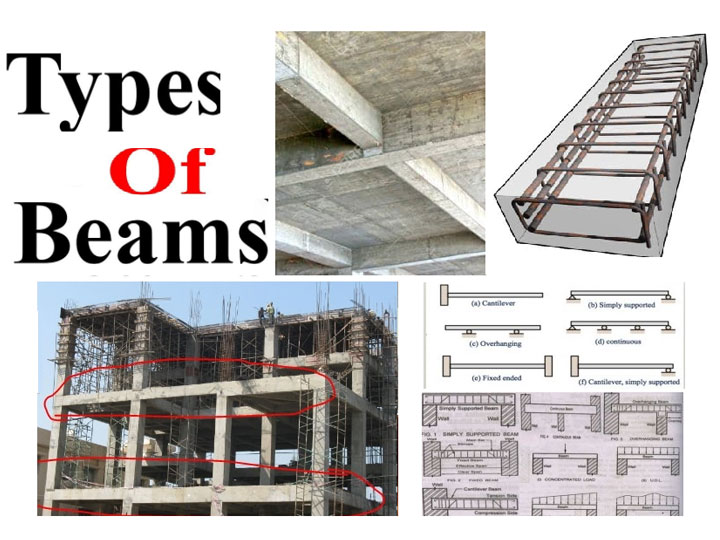Home Multimedia - Videos Beam, Types, Usage, How to Calculate

# Beam, Types, Usage, How to Calculate

In building construction, a beam is a component of structure which resists the load of the beam's axis. Beam is also called a lintel.

## Types of beam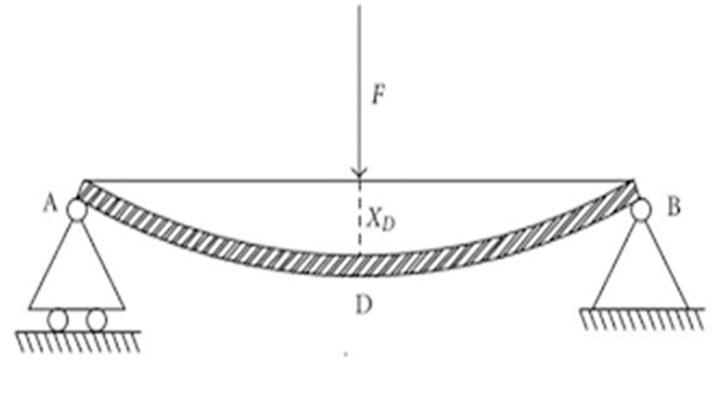### Simply supported beam

This type of beam is supported at its both ends is known as simply supported beam. This is used mainly in 2 to 3 storeyed buildings.

### Cantilever beam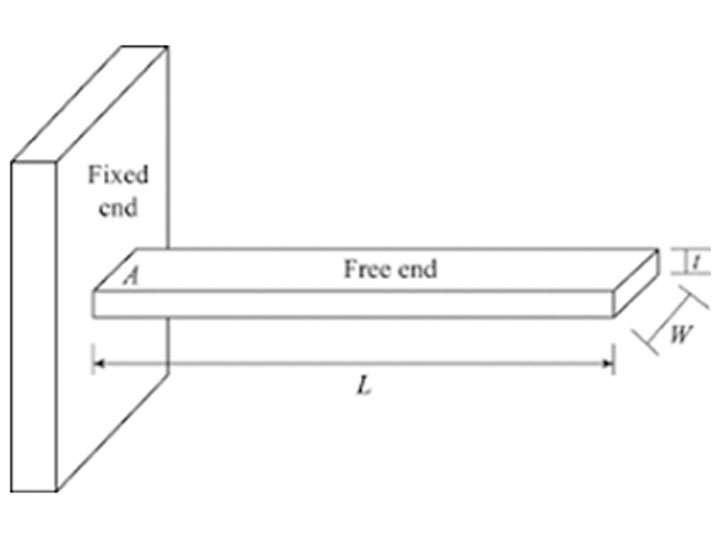This type of beam is fixed at one end and free at the other end is also known as cantilever beam. This beam is used mainly for supporting the sunshade of a bigger span of the building. This type of beam is used for the maximum shear forces & moments developed at the support section, that is a reinforced concrete column.

### Overhanging beam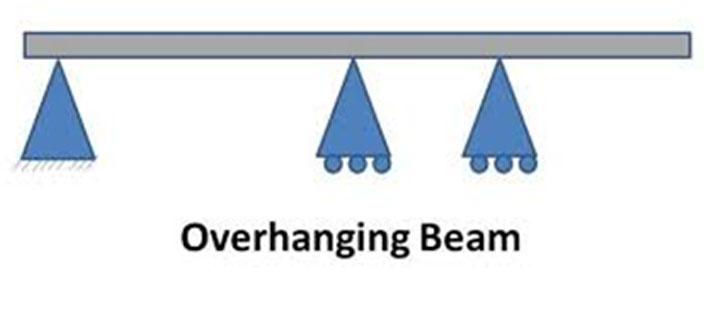In this type of beam the end part of the beam is stretched beyond the support then it is called overhanging beam.

### Fixed beam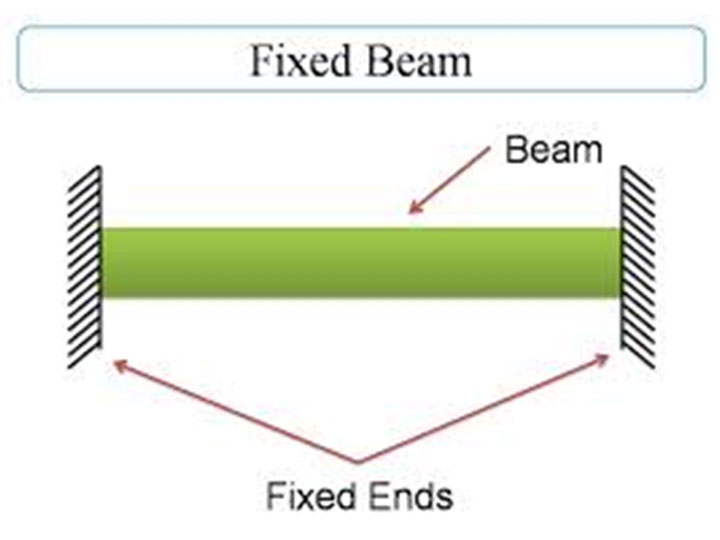In this type of beam, the both ends are fixed in walls, also known as fixed beam.

### Continuous beam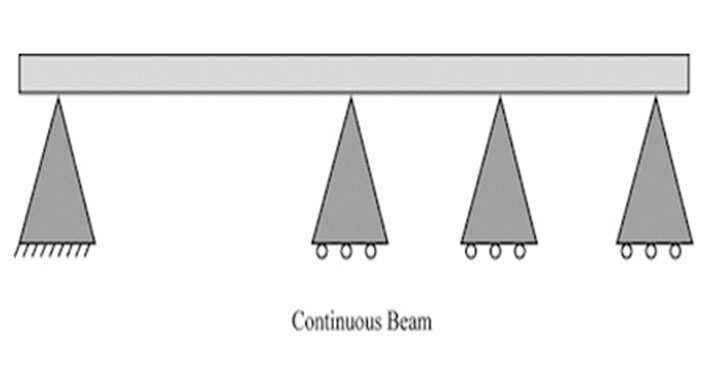This type of beam provides more than two supports known as continuous beam. This type of beam is mainly used in multi storeyed buildings.

### Propped cantilever beam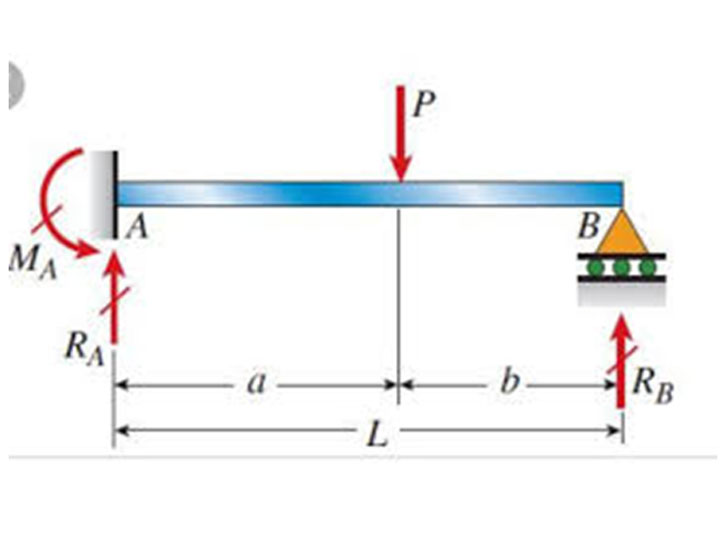In this type of beam the free end is placed on a roller support. It is a modification of a cantilever beam.

### Lintel beam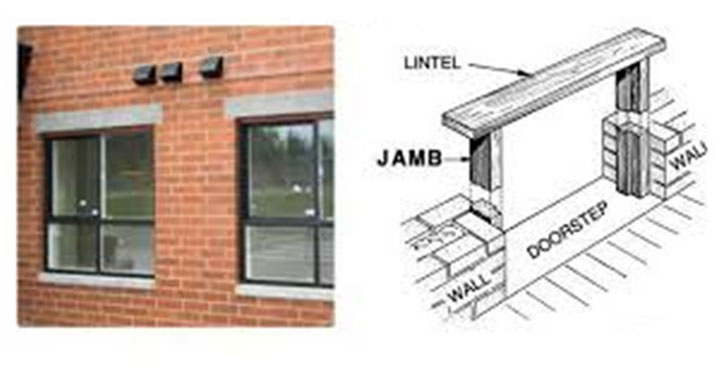This type of beam is used to open the windows and doors. This type of beam behaves like a guard of windows.

There is another type of beam in construction.

• According to the cross section.
1. I beam
2. T beam
• According to geometry
1. Curved beam
2. Tapper beam

## Usage of beam

Beam bear the vertical gravitational force. They also carry the horizontal loads. Beam is also very useful for taking bending loads. The cross section of beam and the orientation of beam is also very helpful. By using beam section workers make the structure stiff by increasing the moment of inertia by disturbing the area away from the neutral axis thus increasing moment of resistance.

## Calculate the beam size

The standard size of the concrete beam at least 230 mm x 230 mm (9" x 9"). According to their span the standard size of the depth of the beam is increased or decreased.

SL NO BEAM NAME SPAN
1 Cantilever 7
2 Simply supported 20
3 continuous 26

Calculation of minimum size of R.C.C beam size as per IS 456: 2000

Effective depth =Span/Basic value

Total Depth = Effective depth + dia./2 + Clear Cover Width = Depth/1.5 (width should not be less than 200 mm)

Note:

As per IS ? 13920,

The width to depth ratio should be more than 0.3.

Width/Depth >0.3

Depth of beam shall not be exceeded ? of the clear span.

#### Example- For simply supported beam

Where,

Le = Effective length
D = Total depth of the beam
d = Effective depth of the beam
b = width of beam

Span of simply supported beam = 5 m
Then effective depth of beam = 5000 / 20
d = 250 mm

Total depth = effective depth + diameter of bar/2 + clear cover

Assume diameter of bar = 16 mm
D = 250 + 16/2 + 25
D = 283 mm ? 285 mm

And width = D/1.5
Width = 285 /1.5
b = 190 mm

So, we will take 200 mm for width

Then,

Width / Depth = 200/ 285 = 0.7 > 0.3, SAFE

Then,
we can check depth of beam = ? of span
= ? x 5000
= 1250 mm > 285 mm, SAFE

#### Example- For a cantilever beam

Span of cantilever beam = 2 m
Then effective depth of beam = 2000 / 7
d = 285 mm
Total depth = effective depth + diameter of bar/2 + clear cover
Assume diameter of bar = 16 mm
D = 285 + 16/2 + 25
D = 318 mm ? 320 mm

And width = D/1.5
Width = 320 /1.5
b = 213 mm

So, we will take 230 mm for width

Then,
Width / Depth = 230/ 320 = 0.71 > 0.3, SAFE

Then,
we can check depth of beam = ? of span
= ? x 2000
= 500 mm > 320 mm, SAFE

Example- For continuous beam

Span of continuous beam = 5 m
Then effective depth of beam = 5000 / 26
d = 192.3 mm ? 200 mm
Total depth = effective depth + diameter of bar/2 + clear cover
Assume diameter of bar = 16 mm
D = 200 + 16/2 + 25
D = 233 mm ? 235 mm

And width = D/1.5
Width = 235 /1.5
b = 156.67 mm

So, we will take 200 mm for width

Then,

Width / Depth = 200/ 235 = 0.85 > 0.3, SAFE

Then,
we can check depth of beam = ? of span
= ? x 5000
= 1250 mm > 235 mm, SAFE

Check for lateral stability or buckling:

(As per IS 456:2000, page no.39, clause 23.3)

For Simply Supported or Continuous Beam

Allowable L = 60 b
Allowable L= 250. b^2 / d
Take the least value of L

If beam span is less than L allowable, then the beam will be safe from lateral stability or buckling.

Where,
b = width of beam
d = effective depth of the beam

Example-
Allowable L = 60 b
Allowable L = 60 x 200
Allowable L = 12000 mm = 12 m
And
Allowable L = 250 b2 / d
Allowable L = 250 x 2002 / 285
Allowable L = 35087.7 mm = 35.087 m

Therefore,
Allowable L = 12 m
Here, Allowable L = 12 m > 5m, SAFE

Thumb rule method

calculate the depth of the beam according to the method

1 foot (span of the beam) = 1Inch (depth of beam)

If the span of the beam is 16 feet, then the depth of the beam will be 16 inches.

To get more details, go through the following video tutorial.